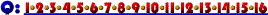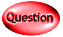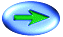5. Economists sometimes find it useful to express demand and supply relationships mathematically, as is done here.

Suppose, as in this question, the market we are interested in can be expressed by the demand equation QD = 40 - 2P and the supply equation QS = 5 + 3P? The question asks "what will happen if the market price is initially \$5?"

As we know, market forces move markets toward equilibrium price and quantity; meaning, if we can determine what the eventual equilibrium for this market will be, we will know in which direction it will move from an initial price of \$5.

In order to determine the equilibrium price and quantity, we simply set supply and demand equal and find the price, P, that solves that equation.

40 - 2P = 5 + 3P

35 = 5P

P* = 7

Therefore, the equilibrium price will eventually be \$7. This means that the price will rise from \$5. As the price rises, the quantity demanded must be falling.